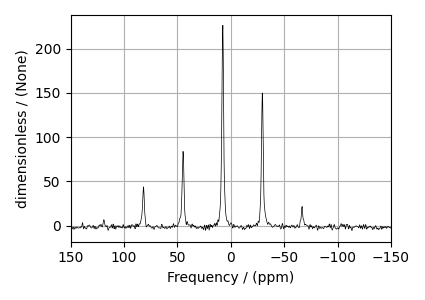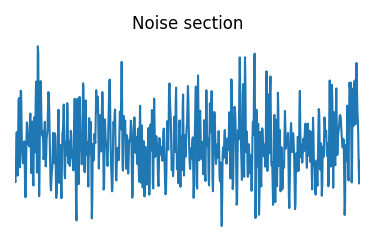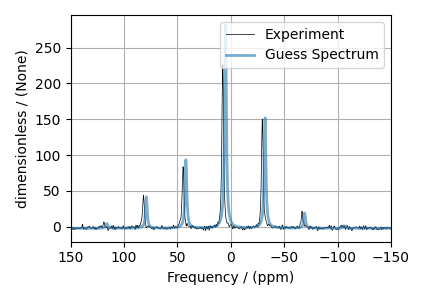# ³¹P MAS NMR of crystalline Na2PO4 (CSA)¶

In this example, we illustrate the use of the mrsimulator objects to

• create a CSA fitting model using Simulator and SignalProcessor objects,

• use the fitting model to perform a least-squares analysis, and

• extract the fitting parameters from the model.

We use the LMFIT library to fit the spectrum. The following example shows the least-squares fitting procedure applied to the $$^{31}\text{P}$$ MAS NMR spectrum of $$\text{Na}_{2}\text{PO}_{4}$$. The following experimental dataset is a part of DMFIT  examples. We thank Dr. Dominique Massiot for sharing the dataset.

Start by importing the relevant modules.

import csdmpy as cp
import matplotlib.pyplot as plt
from lmfit import Minimizer

from mrsimulator import Simulator, SpinSystem, Site
from mrsimulator.method.lib import BlochDecaySpectrum
from mrsimulator import signal_processor as sp
from mrsimulator.utils import spectral_fitting as sf
from mrsimulator.utils import get_spectral_dimensions
from mrsimulator.spin_system.tensors import SymmetricTensor


## Import the dataset¶

Import the experimental data. We use dataset file serialized with the CSDM file-format, using the csdmpy module.

host = "https://nmr.cemhti.cnrs-orleans.fr/Dmfit/Help/csdm/"
filename = "31P Phosphate 6kHz.csdf"

# standard deviation of noise from the dataset
sigma = 1.523217

# For spectral fitting, we only focus on the real part of the complex dataset
experiment = experiment.real

# Convert the dimension coordinates from Hz to ppm.
experiment.x.to("ppm", "nmr_frequency_ratio")

# plot of the dataset.
plt.figure(figsize=(4.25, 3.0))
ax = plt.subplot(projection="csdm")
ax.plot(experiment, color="black", linewidth=0.5, label="Experiment")
ax.set_xlim(150, -150)
plt.grid()
plt.tight_layout()
plt.show()## Create a fitting model¶

A fitting model is a composite of Simulator and SignalProcessor objects.

Step 1: Create initial guess sites and spin systems

P_31 = Site(
isotope="31P",
isotropic_chemical_shift=5.0,  # in ppm,
shielding_symmetric=SymmetricTensor(zeta=-80, eta=0.5),  # zeta in Hz
)

spin_systems = [SpinSystem(sites=[P_31])]


Step 2: Create the method object. Create an appropriate method object that closely resembles the technique used in acquiring the experimental dataset. The attribute values of this method must meet the experimental conditions, including the acquisition channels, the magnetic flux density, rotor angle, rotor frequency, and the spectral/spectroscopic dimension.

In the following example, we set up a Bloch decay spectrum method where the spectral/spectroscopic dimension information, i.e., count, spectral_width, and the reference_offset, is extracted from the CSDM dimension metadata using the get_spectral_dimensions() utility function. The remaining attribute values are set to the experimental conditions.

# get the count, spectral_width, and reference_offset information from the experiment.
spectral_dims = get_spectral_dimensions(experiment)

MAS = BlochDecaySpectrum(
channels=["31P"],
magnetic_flux_density=9.395,  # in T
rotor_frequency=6000,  # in Hz
spectral_dimensions=spectral_dims,
experiment=experiment,  # experimental dataset
)

# A method object queries every spin system for a list of transition pathways that are
# relevant to the given method. Since the method and the number of spin systems remains
# unchanged during the least-squares analysis, a one-time query is sufficient. To avoid
# querying for the transition pathways at every iteration in a least-squares fitting,
# evaluate the transition pathways once and store it as follows
for sys in spin_systems:
sys.transition_pathways = MAS.get_transition_pathways(sys)


Step 3: Create the Simulator object and add the method and spin system objects.

sim = Simulator(spin_systems=spin_systems, methods=[MAS])
sim.run()


Step 4: Create a SignalProcessor class object and apply the post-simulation signal processing operations.

processor = sp.SignalProcessor(
operations=[
sp.IFFT(),
sp.apodization.Exponential(FWHM="0.3 kHz"),
sp.FFT(),
sp.Scale(factor=300),
sp.baseline.ConstantOffset(offset=-2),
]
)
processed_dataset = processor.apply_operations(dataset=sim.methods.simulation).real


Step 5: The plot of the dataset and the guess spectrum.

plt.figure(figsize=(4.25, 3.0))
ax = plt.subplot(projection="csdm")
ax.plot(experiment, color="black", linewidth=0.5, label="Experiment")
ax.plot(processed_dataset, linewidth=2, alpha=0.6, label="Guess Spectrum")
ax.set_xlim(150, -150)
plt.legend()
plt.grid()
plt.tight_layout()
plt.show()## Least-squares minimization with LMFIT¶

Once you have a fitting model, you need to create the list of parameters to use in the least-squares minimization. For this, you may use the Parameters class from LMFIT, as described in the previous example. Here, we make use of a utility function, make_LMFIT_params(), to simplifies the LMFIT parameters generation process. By default, the function only creates parameters from the SpinSystem and SignalProcessor objects. Often, in spectrum with sidebands, spinning speed may not be accurately known; and is, therefore, included as a fitting parameter. To include a keyword from the method object, use the include argument of the function, as follows,

Step 6: Create a list of parameters.

params = sf.make_LMFIT_params(sim, processor, include={"rotor_frequency"})


The make_LMFIT_params parses the instances of the Simulator and the PostSimulator objects for parameters and returns a LMFIT Parameters object.

Customize the Parameters: You may customize the parameters list, params, as desired. Here, we remove the abundance parameter.

params.pop("sys_0_abundance")
print(params.pretty_print(columns=["value", "min", "max", "vary", "expr"]))

Name                                       Value      Min      Max     Vary     Expr
SP_0_operation_1_Exponential_FWHM            0.3     -inf      inf     True     None
SP_0_operation_3_Scale_factor                300     -inf      inf     True     None
SP_0_operation_4_ConstantOffset_offset        -2     -inf      inf     True     None
mth_0_rotor_frequency                       6000     5900     6100     True     None
sys_0_site_0_isotropic_chemical_shift          5     -inf      inf     True     None
sys_0_site_0_shielding_symmetric_eta         0.5        0        1     True     None
sys_0_site_0_shielding_symmetric_zeta        -80     -inf      inf     True     None
None


Step 7: Perform the least-squares minimization. For the user’s convenience, we also provide a utility function, LMFIT_min_function(), for evaluating the difference vector between the simulation and experiment, based on the parameters update. You may use this function directly as the argument of the LMFIT Minimizer class, as follows,

minner = Minimizer(sf.LMFIT_min_function, params, fcn_args=(sim, processor, sigma))
result = minner.minimize()
result


## Fit Statistics

 fitting method leastsq # function evals 99 # data points 2048 # variables 7 chi-square 2364.72004 reduced chi-square 1.15860855 Akaike info crit. 308.494070 Bayesian info crit. 347.866403

## Variables

name value standard error relative error initial value min max vary
sys_0_site_0_isotropic_chemical_shift 7.57609591 0.00347983 (0.05%) 5.0 -inf inf True
sys_0_site_0_shielding_symmetric_zeta -88.1880932 0.20973028 (0.24%) -80.0 -inf inf True
sys_0_site_0_shielding_symmetric_eta 0.45591108 0.01141672 (2.50%) 0.5 0.00000000 1.00000000 True
mth_0_rotor_frequency 6012.45936 0.80710569 (0.01%) 6000.0 5900.00000 6100.00000 True
SP_0_operation_1_Exponential_FWHM 0.27280282 0.00163466 (0.60%) 0.3 -inf inf True
SP_0_operation_3_Scale_factor 268.854046 1.20048569 (0.45%) 300.0 -inf inf True
SP_0_operation_4_ConstantOffset_offset -1.65291839 0.03734295 (2.26%) -2.0 -inf inf True

## Correlations (unreported correlations are < 0.100)

 SP_0_operation_1_Exponential_FWHM SP_0_operation_3_Scale_factor 0.6643 sys_0_site_0_shielding_symmetric_zeta sys_0_site_0_shielding_symmetric_eta 0.362 SP_0_operation_3_Scale_factor SP_0_operation_4_ConstantOffset_offset -0.2423 sys_0_site_0_shielding_symmetric_eta SP_0_operation_3_Scale_factor 0.2274 sys_0_site_0_shielding_symmetric_zeta SP_0_operation_3_Scale_factor -0.2254 sys_0_site_0_isotropic_chemical_shift mth_0_rotor_frequency 0.2033 SP_0_operation_1_Exponential_FWHM SP_0_operation_4_ConstantOffset_offset -0.161 sys_0_site_0_shielding_symmetric_zeta mth_0_rotor_frequency -0.1084

Step 8: The plot of the fit, measurement, and residuals.

# Best fit spectrum
best_fit = sf.bestfit(sim, processor).real
residuals = sf.residuals(sim, processor).real

plt.figure(figsize=(4.25, 3.0))
ax = plt.subplot(projection="csdm")
ax.plot(experiment, color="black", linewidth=0.5, label="Experiment")
ax.plot(residuals, color="gray", linewidth=0.5, label="Residual")
ax.plot(best_fit, linewidth=2, alpha=0.6, label="Best Fit")
ax.set_xlabel(r"$^{31}$P frequency / ppm")
ax.set_xlim(150, -150)
plt.legend()
plt.grid()
plt.tight_layout()
plt.show()Total running time of the script: ( 0 minutes 2.589 seconds)

Gallery generated by Sphinx-Gallery